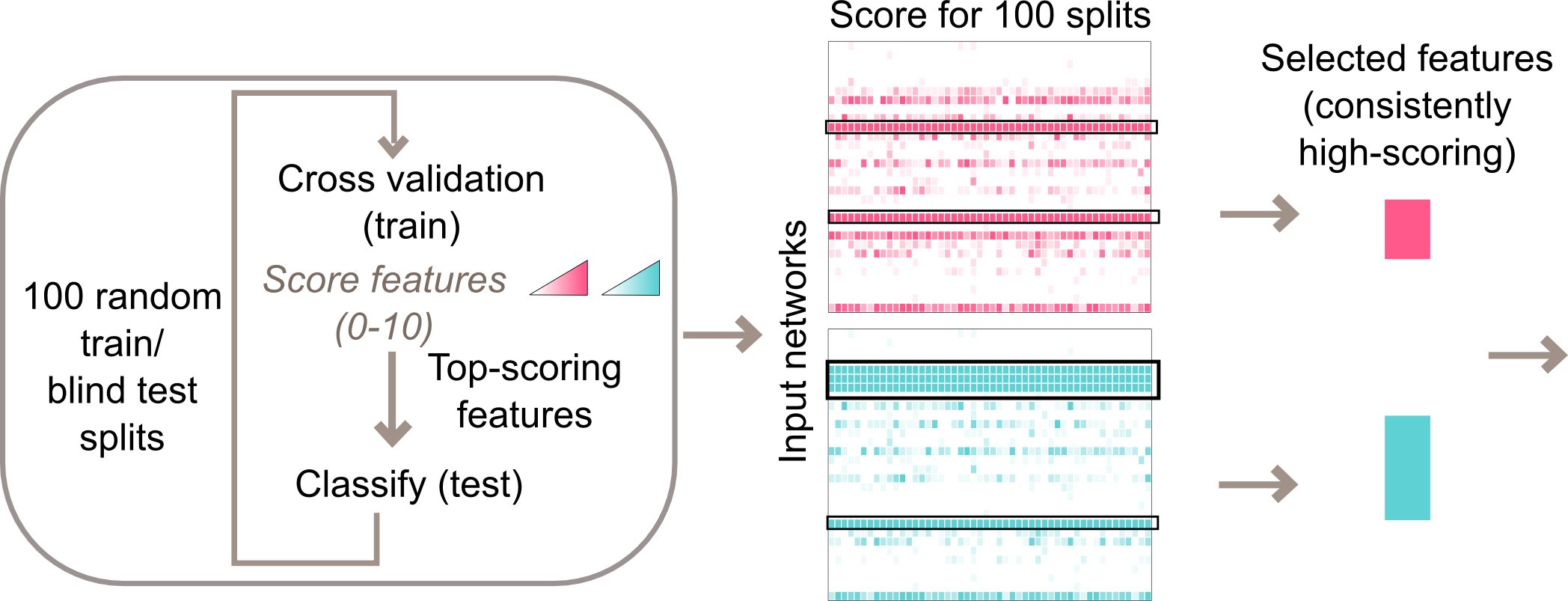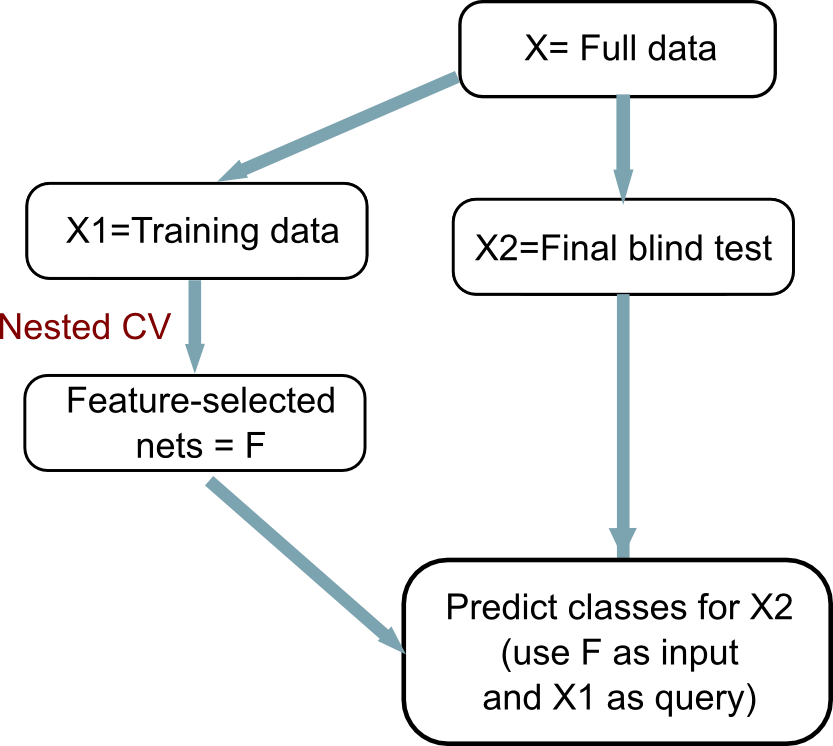# 5. Design the Predictor¶

## 5.1. Nested cross-validation design¶

We recommend a nested cross-validation design for predictor building. Inner loop: Patient samples are split (e.g. 80:20) into a training and a blind test set. Using only the training samples, 10-fold cross validation is performed for each class, generating for each network a score between 0 and 10. Networks that scored 9 or 10 out of 10 are used to classify blind test samples. Note that this loop also generates a performance score for this particular blind test sample.

Outer loop: The inner loop is repeated (e.g. 100 times), which each loop using a new random split of train and blind test (100 trials).

The average of blind test classification across the 100 splits is used to measure overall predictor performance.

Feature-selected networks are those that score consistently well across all the outer loops. For example, a strict criterion would require networks to score score 10 out of 10 in all loops.nestedCV.png Example of nested cross-validation design in binary classification. Here an inner loop of 10-fold cross validation is run 100 times over different splits of data into train and blind test. At the end of the process, each class has a network score table. This matrix (call it `S`) has K rows (where K is the number of networks) and 100 columns. `S[i,j]` has the cross-validation score for network `i` in loop `j`. The user then applies a cutoff to call feature-selected networks.

## 5.2. Overall workflow¶

Ideally a dataset would have sufficient samples to be set aside at the outset, before nested CV (figure below). Comparing predictor performance from the nested CV to that with the final blind test serves as a validation test.workflow_predictor.png